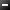Jinan Amrta Air Conditioning Co.,Ltd

Home > News > Choosing A Water Chiller
Product Categories
•Water Chiller
•Air Conditioner
•Heat Pump System
•Fan Coil
•Air Handling Systems
Online Service
News

## Choosing A Water Chiller

How To Choose An Industrial Water Chiller
Choosing the right size recirculating chiller adds to the economies of its use. The optimum size needed is based on the amount of heat your application is generating, plus additional power to maintain temperature under varying loads.

Normally the manufacturer of the equipment you are cooling will supply heat removal information, which will include BTU/hr or watts to be removed along with flow rate and desired and inlet and outlet temperatures for the equipment.

If information isnt available, heres how to calculate the heat load of your system:

BTU/hr = (T1-T2) x gpm x 60 min/hr x 8.33 lb/gal x Cp

T1 = temperature of coolant leaving the equipment, deg F

T2 = temperature of coolant entering the equipment, deg F

gpm = gallons per minute of coolant flowing through the equipment

Cp = specific heat of coolant; Water = 1.0

Measure temperature with the same thermometer if possible of with two thermometers of known accuracy. Measure gpm using a flowmeter of by collecting the coolant in a known volume for a given period of time.

If ambient temperature of the cooling location is above 68°F, add 1% to the calculated BTU/hr for each 0.9°F above 68°F.
If operating at 50Hz, add 20% to the calculated BTU/hr.
If line voltage is consistently below rated voltage, or if you work at high altitude, add 10% to the calculated wattage.
Future growth cooling needs or variability of heat output of existing unit.

Conversions

Watts = BTU/hr / 3.413
Tons = (BTUs / hr) / 12,000

How To Choose A Laboratory Water Chiller
Choosing the right size recirculating chiller adds to the economies of its use. The optimum size needed is based on the amount of heat your application is generating, plus additional power to maintain temperature under varying loads. Normally the manufacturer of the device you are cooling will supply heat removal information. If information isnt available, heres how to calculate the heat load of your system:

Watts
= [DT° x (K)] / S

Where
:
DT= The difference (D) between incoming and outgoing tap water temperature (T) of your instrument. Measure carefully using the same thermometer for both locations. You may measure in Celsius or Fahrenheit.
S = The number of seconds to fill a one liter container.
K = Conversion constant for density and specific heat of water.

Measured in
:
Celsius: Watts = [DT°C (4,186)] / Seconds
Fahrenheit: Watts = [DT°F (2,326)] / Seconds

:
If ambient temperature of the cooling location is above 20°C, add 1% to the calculated wattage for each 0.5°C above 20°C.
If operating at 50Hz, add 20% to the calculated wattage.
If line voltage is consistently below rated voltage, or if you work at high altitude, add 10% to the calculated wattage.
Future growth cooling needs or variability of heat output of existing unit.

Conversions
:
BTUs / hr = (watts) * 3.413
Tons = (BTUs / hr) / 12,000
Below are some basic methods for calculating the heat load of various industrial processes. In order to use the heat load calculations some general definitions need to be addressed. The calculations will reference the following basic definitions and formulas:

One Ton of Refrigeration = 12,000 Btu per Hour

One Refrigeration Ton = 3,025 kg calories per hour

Btu/hr for Water = GPM x 500 x Delta-T

Btu/hr for other fluids = Lbs. Per Hr. x Specific Heat x Specific Gravity X Delta-T

Btu/hr for Solids = Lbs. Per hour x Specific Heat x Delta-T

Btu/hr = kW x 3,413

Btu/hr = HP x 2,544

PSIA = PSIG 14.7

Btu/hr = kW x 1000 / .293

kW = Btu/hr / 1000 x .293

Lbs/Hr = GPM x Density x 8.022

Lbs/Hr = GPM x 501.375 x Specific Gravity

Specific Gravity = Density / 62.4

GPM of Water = Btu/hr / Specific Heat / Specific Gravity / Delta-T / 500

Heat Rejection for Common Industrial Machinery
Air Compressors 1,500 Btu/hr per HP
Air Compressor Aftercooler1,500 Btu/hr per HP
Vacuum Pump Cooling1,500 Btu/hr per HP
Hydraulic Cooling2,544 Btu/hr per HP x .6
Hot Runner..3,420 Btu/hr pr kW

If component heat loads cannot be learned from customer supplied data, multiply the total input Hp or kW times the appropriate conversion factor. This represents the maximum possible heat load.
Share to: